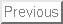Glossary.
• Auxiliary problem. The boundary value problem that defines a Green's function, which includes a differential equation with an impulsive heating term and homogeneous boundary conditions. See What is a Green's Function?''
• Boundary condition. A specified value (or relation) at a surface of a body. See Dirichlet, Neumann, and convection conditions.
• Cartesian coordinates. See rectangular coordinates.
• Causality relation. The requirement that the transient GF is zero for any time before the heat pulse is released: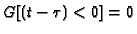. In control theory, a function is said to be causal'' if the response only appears after the effect (heat pulse) that causes it.
• Conductivity. See thermal conductivity.
• Convection at boundary, described by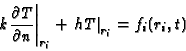Here h is the heat transfer coefficient and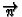is the outward normal at the body surface. The homogeneous convection boundary condition is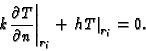• Delta function. See Dirac delta function.
• Dirac delta function. Symbol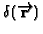, is zero everywhere except at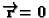in such a way that the integral over the volume is unity. Also called the unit impulse function. See Properties of Dirac delta function.
• Dirichlet boundary condition. Specified temperature at a boundary. The homogeneous Dirichlet boundary condition is T(r i,t)=0.
• energy generation. Symbol g, units [Watts/meter3 or Joule/meter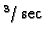], energy deposited in a body per unit volume per unit time, for example from electric heating, microwave absorption, nuclear reaction, etc.
• erf See error function.
• erfc See error function (complementary).
• Error function. Symbol erf(z), is defined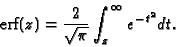• Error function (complementary). Related to the error function by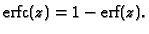• Fin. A solid body exposed to a fluid for the purpose of exchanging heat with the fluid.
• Green's function. A fundamental solution of a linear differential equation satisfying homogeneous boundary conditions. (other names include influence function, impulse response, source solution). See What is Green's Function?
• Green's function solution equation. Formal solution to a boundary value problem in the form of one or more integrals, each of which contains a Green's function and a non-homogeneous term (driving term''). The non-homogeneous terms may be boundary conditions, initial conditions, or volume energy generation.
• Heat equation. Also called the transient heat conduction equation. Describes the movement of heat by diffusion (molecule-to-molecule transport) in a solid (or motionless) medium. In vector notation,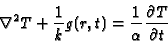Here T is temperature, k is conductivity, g is energy generation,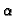is thermal diffusivity, r is spatial coordinate, and t is time.
• Heat flux. Energy per unit time per unit area. Units Watts/meters2.
• Heat transfer coefficient. Symbol h, units [W/m2/K]. Relates the surface temperature and surface heat flux with a surrounding fluid according to Newton's law of cooling:

qsurface=h(Tsurface-Tfluid) .

• Helmholtz equation. Given by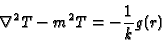When m2 is real this equation describes steady heat with side'' heat losses (fin losses). When m2 is imaginary the Helmholtz equation is the heat equation in Fourier-transform space (also called the thermal-wave equation). Finally, replace -m2 by real +m2 to give the wave equation in Fourier-transform space.
• Homogeneous equation. An equation in which every non-zero term contains the independent variable. For example, in a homogeneous heat equation every term contains T (there is no energy generation term).
• Homogeneous boundary condition. A boundary condition defined by an equation in which the temperature appears in every non-zero term. The following boundary conditions are homogeneous:
type 1, T = 0;
type 2,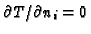;
type 3,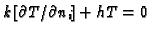.
• Homogeneous body. A body composed of the same material all the way through.
• Initial condition. Temperature distribution at t=0.
• Insulated boundary. A boundary with no heat flow, defined byon the boundary. See also Neumann boundary condition.
• Laplace equation. Also called the steady heat conduction equation.
• Multiplicative property. For transient heat conduction, many rectangular and cylindrical 2-D and 3-D GF may be constructed by multiplying 1-D GF. See Beck (1992, section 4.5) for restrictions.
• Neumann boundary condition. The specified heat flux boundary condition, defined by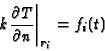whereis the outward normal on the body surface at ri. The homogeneous Neumann condition is the insulated boundary,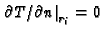.
• Pseudo Green's function. A GF modified for use in a body with all boundaries of type 2 (specified heat flux); for these problems the usual GF does not exist. The defining auxiliary equation for the pseudo GF has an additional term.
• Rectangular coordinates. Cartesian coordinates (x,y,z).
• Reciprocity. A GF that is symmetric with respect to space and asymmetric with respect to time is said to exhibit reciprocity. see Properties of GF.
• Specific heat. Material property with symbol c and units [Joule/kg/K]. The amount of energy needed to raise a unit mass of a material one degree.
• Spherical coordinates. also called spherical polar coordinates,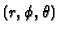.
• Temperature. Symbol T, units Kelvin. A measure of the intensity of thermal energy present in a body.
• Thermal diffusivity. Symbol, units m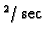. A defined property found from specific heat, density and thermal conductivity: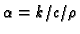.
• Thermal conductivity. Symbol k, units W/m/K. Defines the proportionality relation between heat flux and temperature gradient, defined by Fourier's law: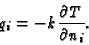• Volume energy generation. See energy generation.
• Watt. A unit of power equal to one Joule per second.